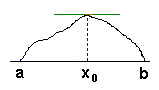# Rolle's Theorem and the Mean Value Theorem

Intermediate Value Theorem and Location Principle both apply to provide information about existence of tangent lines or, which is the same, derivatives of functions. What results is two formulations of the Mean Value Theorem of which one is more general but both are equivalent.### Theorem 1 (T1, Rolle's Theorem)

Let, for two real a and b, a < b, a function f be differentiable on a closed interval [a, b] such that f(a) = f(b) = 0. Then there exists a number x0[a, b] such that the line tangent to the graph of f at the point x0 is horizontal, i.e. f'(x0) = 0.### Theorem 2 (T2, Mean Value Theorem)

Let, for two real a and b, a < b, a function f be differentiable on a closed interval [a, b]. Then there exists a number x0[a, b] such that the line tangent to the graph of f at the point x0 is parallel to the chord connecting the two end points of the graph - (a, f(a)) and (b, f(b)).Clearly T1 is only a special case of T2. However it's interesting that the more general T2 could be deduced from its special case, T1. Introduce a new function

(2)
 g(x) = f(x) - (f(a) + (x - a)·(f(b) - f(a))/(b - a)).

Then it follows that g(a) = f(a) - (f(a) + 0) = 0 whereas

(2)
 g(b) = f(b) - (f(a) + (b - a)·(f(b) - f(a))/(b - a) = f(b) - (f(a) + (f(b) - f(a)) = 0.

Therefore, g(a)=g(b)=0 and we can apply T1 since g is also continuously differentiable on [a, b]. Therefore, there exists x0[a, b] such that g'(x0) = 0. Written explicitly this says f'(x) - (f(b) - f(a))/(b - a) = 0.

Q.E.D.

(There is a second, more extended, attempt, on this topic that is accompanied by an interactive Java illustration.)